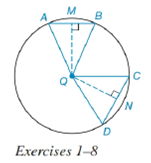Chapter 6.4, Problem 2EElementary Geometry For College St...

7th Edition
Alexander + 2 others
ISBN: 9781337614085

Solutions

Chapter
SectionElementary Geometry For College St...

7th Edition
Alexander + 2 others
ISBN: 9781337614085
Textbook Problem

In Exercises 1 to 8, use the figure provided.If m C D ⌢ < m A B ⌢ , write an inequality that compares C D and A B .To determine

To write:

An inequality that compares CD and AB.

Explanation

Given:

mCD<mAB and the figure given below

Theorem:

In a circle (or in congruent circles) containing two unequal minor arcs, the greater minor arc corresponds to the longer of the chords related to these arcs.

Calculation:

Using the theorem.

Since the arcs are unequal i.e. mCD<mAB and from the given figure

Still sussing out bartleby?

Check out a sample textbook solution.

See a sample solution

The Solution to Your Study Problems

Bartleby provides explanations to thousands of textbook problems written by our experts, many with advanced degrees!

Get Started

Find more solutions based on key concepts